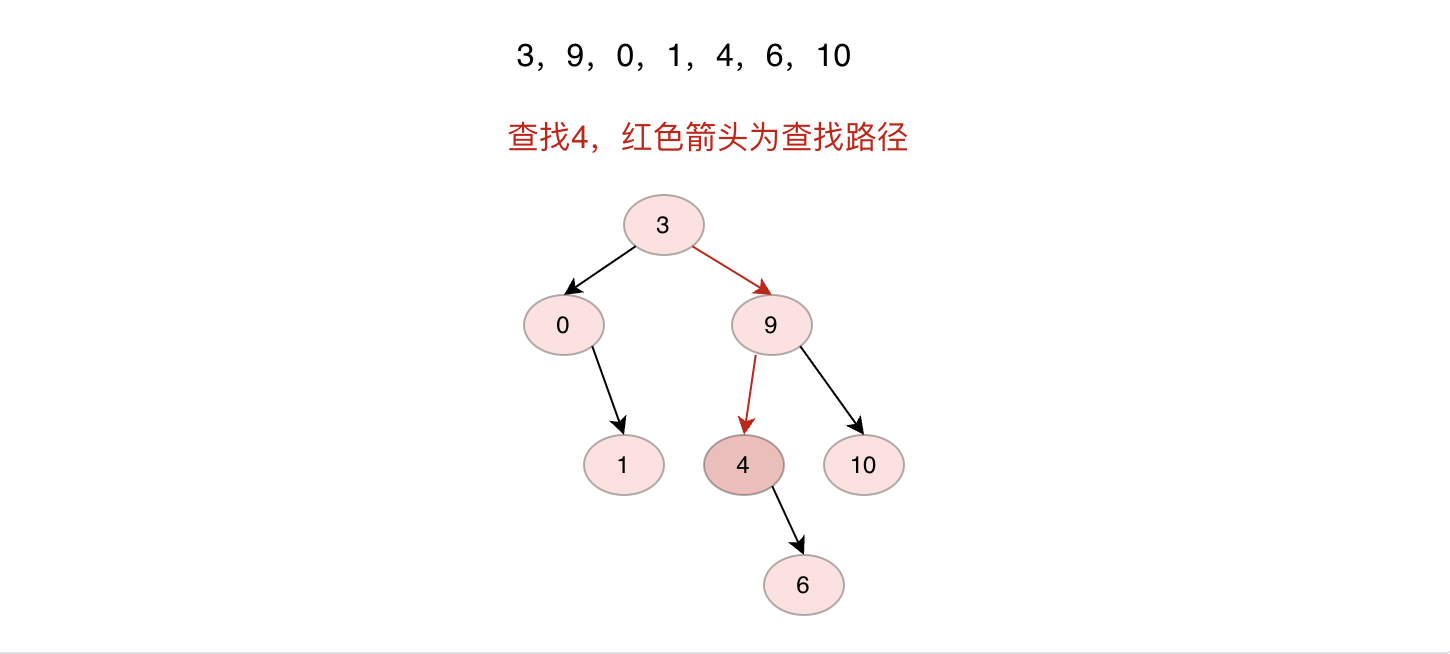﻿ Java数据结构之二叉搜索树详解_java_脚本之家
java# Java数据结构之二叉搜索树详解

## 性质## 实现

### 节点结构

```public class TreeNode {
public int val;
public TreeNode left;
public TreeNode right;

public TreeNode() {
}

public TreeNode(int val) {
this.val = val;
}

public TreeNode(int val, TreeNode left, TreeNode right) {
this.val = val;
this.left = left;
this.right = right;
}
}
```

### 初始化

```TreeNode head;
private void init() {
//添加一个头节点
}
```

### 插入节点```/**
* 插入新节点，假设新节点均大于0
* @param val 插入节点值
* @return 插入的节点
*/
public TreeNode insert(int val) {
while (true) {
if (temp.val < val) {
//val应该在右子树上
if (null != temp.right) {
temp = temp.right;
continue;
} else {
temp.right = new TreeNode(val);
return temp.right;
}
}
//应该在左子树上
if (null != temp.left) {
temp = temp.left;
continue;
}
temp.left = new TreeNode(val);
return temp.left;
}
}
```

### 查找节点```/**
* 搜索节点值
* @param val
* @return
*/
public Pair<TreeNode, TreeNode> find(int val) {
while (null != temp) {
if (temp.val == val) {
return Pair.of(temp, parent);
}
parent = temp;
if (temp.val < val) {
//在右子树上
temp = temp.right;
continue;
}
temp = temp.left;
}
return null;
}
```

### 删除节点

1.如果删除的是叶子节点直接删除2.如果删除的节点只有左子树或者右子树，则直接将左子树或者右子树节点放在删除节点位置3.如果删除节点同时有左子树和右子树，则将右子树节点放在原来节点位置，将左子树放在右子树最左边节点左子树上（反之将左子树放在原来节点位置，右子树放在左子树最右边节点右子树上也可）```/**
* 1.如果删除的是叶子节点直接删除，
* 2.如果删除的节点只有左子树或者右子树，则直接将左子树或者右子树节点放在删除节点位置
* 3.如果删除节点同时右左子树和右子树，则将右子树节点放在原来节点位置，将左子树放在右子树最左边节点左子树上
* @param val
*/
public void delete(int val) {
//找到删除节点，删除节点父节点
Pair<TreeNode, TreeNode> curAndParent = this.find(val);
TreeNode cur = curAndParent.getLeft();
TreeNode parent = curAndParent.getRight();
//记录删除当前节点后，当前节点位置放置哪个节点
TreeNode changed;
if (null == cur.left && null == cur.right) {
changed = null;
} else if (null != cur.left && null != cur.right) {
TreeNode tempRight = cur.right;
while (null != tempRight.left) {
//找到最左侧节点
tempRight = tempRight.left;
}
tempRight.left = cur.left;
changed = cur.right;
} else if (null != cur.left) {
changed = cur.left;
} else {
changed = cur.right;
}
if (parent.left == cur) {
parent.left = changed;
return;
}
parent.right = changed;
}
```